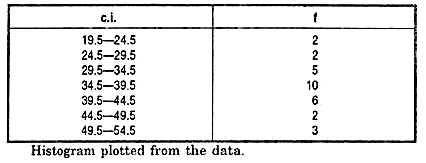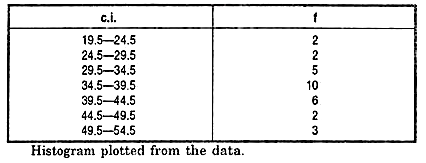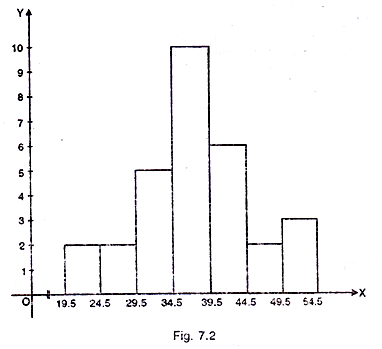# Graphical Representation of Data: Appropriate Usages of Bar Chart, Pie Charts, Histogram

Graphic representation is another way of analyzing numerical data. A graph is a sort of chart through which statistical data are represented in the form of lines or curves drawn across the coordinated points plotted on its surface.

Graphs enable us in studying the cause and effect relationship between two variables. Graphs help to measure the extent of change in one variable when another variable changes by a certain amount.

Graphs also enable us in studying both time series and frequency distribution as they give clear account and precise picture of problem. Graphs are also easy to understand and eye catching.

General Principles of Graphic Representation

There are some algebraic principles which apply to all types of graphic representation of data. In a graph there are two lines called coordinate axes. One is vertical known as Y axis and the other is horizontal called X axis. These two lines are perpendicular to each other. Where these two lines intersect each other is called ‘0’ or the Origin. On the X axis the distances right to the origin have positive value (see fig. 7.1) and distances left to the origin have negative value. On the Y axis distances above the origin have a positive value and below the origin have a negative value.### BAR CHART

A bar chart or bar graph is a chart or graph that presents categorical data with rectangular bars with heights or lengths proportional to the values that they represent. The bars can be plotted vertically or horizontally. A vertical bar chart is sometimes called a line graph.

A bar graph shows comparisons among discrete categories. One axis of the chart shows the specific categories being compared, and the other axis represents a measured value. Some bar graphs present bars clustered in groups of more than one, showing the values of more than one measured variable.

A vertical bar graph is shown below:

Number of students went to different states for study:The rectangular bars are separated by some distance in order to distinguish them from one another. The bar graph shows comparison among the given categories.

Mostly, horizontal axis of the graph represents specific categories and vertical axis shows the discrete numerical values.

### PIE CHART

A pie chart (or a circle chart) is a circular statistical graphic, which is divided into slices to illustrate numerical proportion. In a pie chart, the arc length of each slice (and consequently its central angle and area), is proportional to the quantity it represents. While it is named for its resemblance to a pie which has been sliced, there are variations on the way it can be presented. The earliest known pie chart is generally credited to William Playfair’s Statistical Breviary of 1801.

Pie charts are very widely used in the business world and the mass media.  However, they have been criticized, and many experts recommend avoiding them, pointing out that research has shown it is difficult to compare different sections of a given pie chart, or to compare data across different pie charts. Pie charts can be replaced in most cases by other plots such as the bar chart, box plot or dot plots.Fig. – Pie chart of populations of English native speakers

### HISTOGRAM

Histogram is a non-cumulative frequency graph, it is drawn on a natural scale in which the representative frequencies of the different class of values are represented through vertical rectangles drawn closed to each other. Measure of central tendency, mode can be easily determined with the help of this graph.

How to draw a Histogram?

Step—1

Represent the class intervals of the variables along the X axis and their frequencies along the Y-axis on natural scale.

Step—2

Start X axis with the lower limit of the lowest class interval. When the lower limit happens to be a distant score from the origin give a break in the X-axis n to indicate that the vertical axis has been moved in for convenience.

Step—3

Now draw rectangular bars in parallel to Y axis above each of the class intervals with class units as base: The areas of rectangles must be proportional to the frequencies of the cor­responding classes.Solution

In this graph we shall take class intervals in the X axis and frequencies in the Y axis. Before plotting the graph we have to convert the class into their exact limits.1. It is easy to draw and simple to understand.
2. It helps us to understand the distribution easily and quickly.
3. It is more precise than the polygene.

Limitations of histogram

1. It is not possible to plot more than one distribution on same axes as histogram.
2. Comparison of more than one frequency distribution on the same axes is not possible.
3. It is not possible to make it smooth.

Uses of histogram

1. Represents the data in graphic form.
2. Provides the knowledge of how the scores in the group are distributed. Whether the scores are piled up at the lower or higher end of the distribution or are evenly and regularly distributed throughout the scale.
3. Frequency Polygon. The frequency polygon is a frequen­cy graph which is drawn by joining the coordinating points of the mid-values of the class intervals and their corresponding fre­quencies.

## 2 thoughts on “Graphical Representation of Data: Appropriate Usages of Bar Chart, Pie Charts, Histogram”

error: Content is protected !!